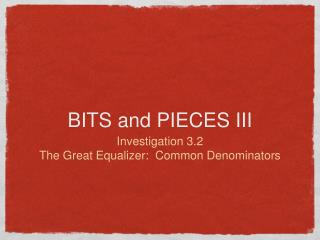DownloadDownload PresentationBITS and PIECES III

# BITS and PIECES III

Télécharger la présentation## BITS and PIECES III

- - - - - - - - - - - - - - - - - - - - - - - - - - - E N D - - - - - - - - - - - - - - - - - - - - - - - - - - -
##### Presentation Transcript

1. BITS and PIECES III • Investigation 3.2 • The Great Equalizer: Common Denominators

2. What does the division 3.25 divided by 0.5 mean?

3. What does the division 3.25 divided by 0.5 mean? How many 0.5’s are in 3.25.

4. What can you give for a real-life situation that this decimal division problem could represent?

5. What can you give for a real-life situation that this decimal division problem could represent? How many half-meter sticks can be cut from a stick 3.25 meters long?

6. What is a reasonable estimate for 3.25 divided by 0.5?

7. What is a reasonable estimate for 3.25 divided by 0.5? 3 divided by 1/2 = 6

8. 3.25 divided by 1/2 ------Actual Answer 3 divided by 1/2 = 6 ------Estimate Is this estimate greater than or less than the actual answer?

9. 3.25 divided by 1/2 ------Actual Answer 3 divided by 1/2 = 6 ------Estimate Is this estimate greater than or less than the actual answer? Less than because you replaced the dividend (3.25) with a less number (3.00) but kept the divisor (1/2) the same.

10. ALL THE SAME 3.25 divided by 0.5 325/100 divided by 5/10 325/100 divided by 50/100

11. 3.25 divided by 0.5 = 6.5 or 6 1/2 What does the quotient 6.5 represent?

12. 3.25 divided by 0.5 = 6.5 or 6 1/2 What does the quotient 6.5 represent? There are 6 whole 0.5’s and half of a 0.5 in 3.25. Can someone dry a picture of this?

13. 3.25 divided by 0.5 = 6.5 or 6 1/2 What does the quotient 6.5 represent? There are 6 whole 0.5’s and half of a 0.5 in 3.25. Can someone dry a picture of this?

14. 4.2 divided by 2.1 Estimate the quotient and write each dividend (4.2) and divisor (2.1) in fraction form with common denominators.

15. 4.2 divided by 2.1 Estimate the quotient and write each dividend (4.2) and divisor (2.1) in fraction form with common denominators. Estimate: 4 divided by 2 =2 Fraction form: 42/10 divided by 21/10 =2 Whole number division: 42 divided by 21

16. 0.44 divided by 0.08 Estimate the quotient and write each dividend and divisor in fraction form with common denominators.

17. 0.44 divided by 0.08 Estimate the quotient and write each dividend and divisor in fraction form with common denominators. Estimate: 0.50 divided by 0.10 = 5 Fraction: 44/100 divided by 8/100 Whole number: 44 divided by 8 = 5.5

18. Write the whole number division problem that corresponds to each problem from each set.

19. Write the whole number division problem that corresponds to each problem from each set.

20. Without solving decide whether each set lead to the same quotient. Explain.

21. Without solving decide whether each set lead to the same quotient. Explain. Set 1 and Set 3 lead to the same answer. The problems in set 2 do not because the quantities being divided are different in each problem. Hint: Move decimals.

22. Solve the problems.

23. Solve the problems.

24. 785/10 divided by 91/10

25. 785 divided by 91

26. -For problem number one just divide to get 25. -For number two, move the decimal point to the right 2 spots to get the answer (25). -For problem three, move the decimal to the right 3 times to get 37500 divided by 15 to get 2500.